# Varieties of Wave Equations

Early in the 20th century, electrons were shown to have wave properties, and the wave-particle duality became a part of our understanding of nature. The mathematics for describing the behavior of such electron waves might be expected to be similar to that for describing classical waves, such as the wave on a stretched string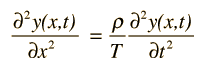or a plane electromagnetic wave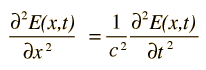The wave equation developed by Erwin Schrodinger in 1926 shows some similarities in its one-dimensional form:Index

Schrodinger equation concepts

 HyperPhysics***** Quantum Physics R Nave
Go Back

# The Postulates of Quantum Mechanics1. Associated with any particle moving in a conservative field of force is a wave function which determines everything that can be known about the system.2. With every physical observable q there is associated an operator Q, which when operating upon the wavefunction associated with a definite value of that observable will yield that value times the wavefunction.3. Any operator Q associated with a physically measurable property q will be Hermitian.4. The set of eigenfunctions of operator Q will form a complete set of linearly independent functions.5. For a system described by a given wavefunction, the expectation value of any property q can be found by performing the expectation value integral with respect to that wavefunction.6. The time evolution of the wavefunction is given by the time dependent Schrodinger equation.
Index

Schrodinger equation concepts

 HyperPhysics***** Quantum Physics R Nave
Go Back

# The Wavefunction Postulate

It is one of the postulates of quantum mechanics that for a physical system consisting of a particle there is an associated wavefunction. This wavefunction determines everything that can be known about the system. The wavefunction is assumed here to be a single-valued function of position and time, since that is sufficient to guarantee an unambiguous value of probability of finding the particle at a particular position and time. The wavefunction may be a complex function, since it is its product with its complex conjugate which specifies the real physical probability of finding the particle in a particular state.### Constraints on the wavefunction

Index

Schrodinger equation concepts

Postulates of quantum mechanics

 HyperPhysics***** Quantum Physics R Nave
Go Back

# Constraints on Wavefunction

In order to represent a physically observable system, the wavefunction must satisfy certain constraints: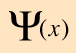Further
discussion

1. Must be a solution of the Schrodinger equation.

2. Must be normalizable. This implies that the wavefunction approaches zero as x approaches infinity.

3. Must be a continuous function of x.

4. The slope of the function in x must be continuous.
 Specificallymust be continuous.

These constraints are applied to the boundary conditions on the solutions, and in the process help determine the energy eigenvalues.

Index

Schrodinger equation concepts

 HyperPhysics***** Quantum Physics R Nave
Go Back

# Probability in Quantum Mechanics

The wavefunction represents the probability amplitude for finding a particle at a given point in space at a given time. The actual probability of finding the particle is given by the product of the wavefunction with its complex conjugate (like the square of the amplitude for a complex function).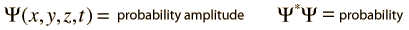Since the probability must be = 1 for finding the particle somewhere, the wavefunction must be normalized. That is, the sum of the probabilities for all of space must be equal to one. This is expressed by the integral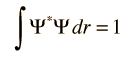Examples of normalization

Part of a working solution to the Schrodinger equation is the normalization of the solution to obtain the physically applicable probability amplitudes.
Index

Schrodinger equation concepts

 HyperPhysics***** Quantum Physics R Nave
Go Back

# Normalization Examples

In order to use the wavefunction calculated from the Schrodinger equation to determine the value of any physical observable, it must be normalized so that the probability integrated over all space is equal to one.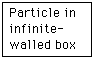Index

Schrodinger equation concepts

 HyperPhysics***** Quantum Physics R Nave
Go Back